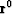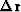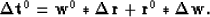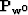Next: Synthetic and real data Up: SIMULTANEOUS ESTIMATION OF WAVELET Previous: Wavelet estimation with a

## Concatenating the two operators

Letandbe some initial estimations of the wavelet and reflectivity sequence respectively. The data residuals can be described bywhich can be linearized for smalland:(15)

By applying theoperator to equation (15), we getIf the sequenceis sufficiently sparse, the last term will involve the correlation betweenand, which we can expect to be somewhat uncorrelated. The same reasoning can be applied to the operator, resulting in the following recursion for reflectivities and waveform(16) (17)

If we start the process with an initial estimation for the wavelet, then the initial estimation for the reflectivity sequence can be obtained when we applyto the trace. As in most non-linear processes, the final solution depends on the initial choice for the wavelet. In all the next examples I used the minimum-phase wavelet corresponding to the smoothed spectrum of the trace as an initial estimation of the wavelet. Although the method has always converged for several different selections of the starting wavelet, an analytical proof that assures its convergence has not yet been derived.Next: Synthetic and real data Up: SIMULTANEOUS ESTIMATION OF WAVELET Previous: Wavelet estimation with a
Stanford Exploration Project
1/13/1998